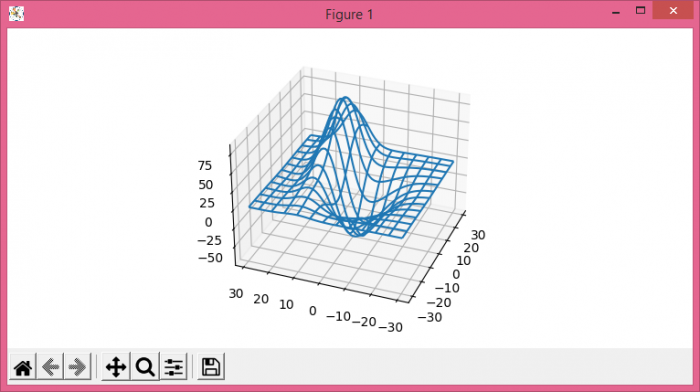# How to save Matplotlib 3d rotating plots?

To save Matplotlib 3d roatating plots, we can take the following steps −

• Set the figure size and adjust the padding between and around the subplots.
• Create a new figure or activate an existing figure.
• Add an '~.axes.Axes' to the figure as part of a subplot arrangement.
• Return a tuple X, Y, Z with a test data set.
• Plot a 3D wireframe.
• Rotate the axis with an angle.
• Redraw the current figure.
• Run the GUI event loop for some seconds.
• To display the figure, use show() method.

## Example

from mpl_toolkits.mplot3d import axes3d
import matplotlib.pyplot as plt

plt.rcParams["figure.figsize"] = [7.50, 3.50]
plt.rcParams["figure.autolayout"] = True

fig = plt.figure()
X, Y, Z = axes3d.get_test_data(0.1)
ax.plot_wireframe(X, Y, Z, rstride=5, cstride=5)

for angle in range(0, 360):
ax.view_init(30, angle)
plt.draw()
plt.pause(.001)

plt.show()

## Output